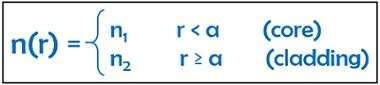# Step Index Fiber

Definition: Step index fiber is a type of optical fibers that holds its classification on the basis of refractive index. Step index fiber is that optical waveguide, that has a constant refractive index within the core and another constant refractive index within the cladding.

The refractive index of the core is somewhat more as compared to that of the cladding. However, it is to be noted here that at the core-cladding interface the refractive index shows sudden variation.

The figure given below shows the step index fiber with its refractive index profile:When light ray propagates along the step index optical fiber then it follows a zigzag path. However, these zigzag patterns are totally composed of straight lines due to total internal reflection.

Mathematically, the refractive index of step index fibre is given as:: a is the core radius

##### Step index single mode fiber

In step index single mode fiber, the core diameter is extremely small, that it allows only one mode to propagate through it. This means that only single light ray propagates through the step index fiber. Due to this the transmitted ray does not experience distortion due to delay differences.

The figure given below shows the propagation of light ray through step index single mode optical fiber:Here we can clearly see that the diameter of the core is extremely narrow. Due to this, only a single mode propagates down the fiber. Here, the core size lies somewhere between 2 to 15 micrometre.

### Step index multimode fiber

In step-index multimode fiber, the diameter of the core is sufficiently large that it allows multiple modes to propagate through it. This simply means that several light rays can propagate down the fiber in case of step-index multimode fiber.

Here, due to the propagation of multiple light rays simultaneously, the fiber experiences distortion due to delay in propagation time.

The figure given below shows the propagation of light rays through step-index multimode optical fiber:Here, the above figure clearly shows that the diameter of the core is large enough that it allows multiple propagating paths. In this case, the core size lies nearly between 50 to 1000 micrometre.

It is to be noted in case of the step index fiber that the variation in index is given as:Majorly the light source used in these fibers is light emitting diodes.

### Advantages of Step Index Fiber

1. The manufacturing of these fibers is quite easy.
2. It is inexpensive.
3. The propagation takes place through total internal reflection.

### Disadvantages of Step Index Fiber

1. In these fibers, as only one light ray propagates through the fiber at a time then it somewhat leads to a drawback in terms of its capacity to carry information signal.
2. Also due to the small diameter of the core, coupling the light inside it is somewhat difficult.

### Applications of Step Index Fiber

These optical fibers majorly find its applications in local area network connections. The reason for this is that it has less information transmitting capacity as compared to graded index fiber.